For CE transistor amplifier, the audio signal voltage across the collector resistance of 2k $\Omega$ is 4 V. If the current amplification factor of the transistor is 100 and the base resistance is I k$\Omega$, then the input signal voltage is

(a) 10 mV                  (b) 20 mV

(c) 30 mV                  (d) 15 mV

Concept Questions :-

Applications of Transistor
High Yielding Test Series + Question Bank - NEET 2020

Difficulty Level:

The given circuit has two ideal diodes connected as shown in the figure below. The current flowing through the resistance ${R}_{1}$ will be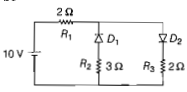(a) 2.5 A                     (b) 10.0 A

(c) 1.43 A                   (d) 3. 13 A

Concept Questions :-

Applications of PN junction
High Yielding Test Series + Question Bank - NEET 2020

Difficulty Level:

What is the output Y in the following circuit, when all the three inputs A,B,C are first 0 and then 1 ?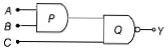1. 0,1                   2. 0,0

3. 1,0                   4. 1,1

Concept Questions :-

Logic gates
High Yielding Test Series + Question Bank - NEET 2020

Difficulty Level:

To get output 1 for the following circuit, the correct choice for the input is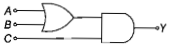(a)        (b)

(c)        (d)

Concept Questions :-

Logic gates
High Yielding Test Series + Question Bank - NEET 2020

Difficulty Level:

A n-p-n transistor is connected in common emitter configuration in a given amplifier. A load resistance of 800Ω is connected in the collector circuit and the voltage drop across it is 0.8 V. If the current amplification factor is 0.96 and the input resistance of the circuits is 192 Ω the voltage gain and the power gain of the amplifier will respectively be

(a)3.69,3.84 (b)4,4 (c)4,3.69 (d)4,3.84

Concept Questions :-

Transistor
High Yielding Test Series + Question Bank - NEET 2020

Difficulty Level:

If in a p-n junction, a square input signal of 10V is applied as shown,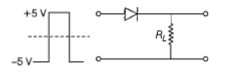then the output across RL will be
(a)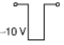(b)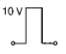(c)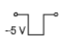(d)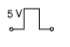Concept Questions :-

Logic gates
High Yielding Test Series + Question Bank - NEET 2020

Difficulty Level:

In the given figure, a diode D is connected to an external resistance R = 100 Ω  and an e.m.f of 3.5 V. If the barrier potential developed across the diode is 0.5 V, the current in the circuit will be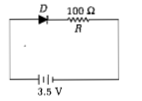1. 30mA

2. 40mA

3. 20mA

4. 35mA

Concept Questions :-

PN junction
High Yielding Test Series + Question Bank - NEET 2020

Difficulty Level:

The input signal given to a CE amplifier having a voltage gain of 150 is Vi=2cos(15t+π/3). The corresponding output signal will be

(a)300cos(15t+π/3)

(b)75cos(15t+2π/3)

(c)2cos(15t+5π/3)

(d)300cos(15t+4π/3)

High Yielding Test Series + Question Bank - NEET 2020

Difficulty Level:

The given graph represents V-I characteristic for a semiconductor device. Which of the following statement is correct?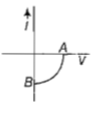(a) It is V-I characteristic for solar cell where point A represents open circuit voltage and point B short circuit current

(b) It is for a solar cell and points A and B represent open circuit voltage and current, respectively

(c) It is for a photodiode and points A and B represent open circuit voltage and current, respectively

(d) It is for a LED and points A and B represents open circuit voltage and short circuit current respectively

Concept Questions :-

Applications of PN junction
High Yielding Test Series + Question Bank - NEET 2020

Difficulty Level:

The barrier potential of a p-n junction depends on

(i)type of semiconductor material

(ii)amount of doping

(iii)temperature

Which one of the following is correct

1. (i) and (ii)only

2. (ii) only

3. (ii) and (iii)only

4. (i),(ii) and (iii)

Concept Questions :-

PN junction